# day 01

## 文件运行过程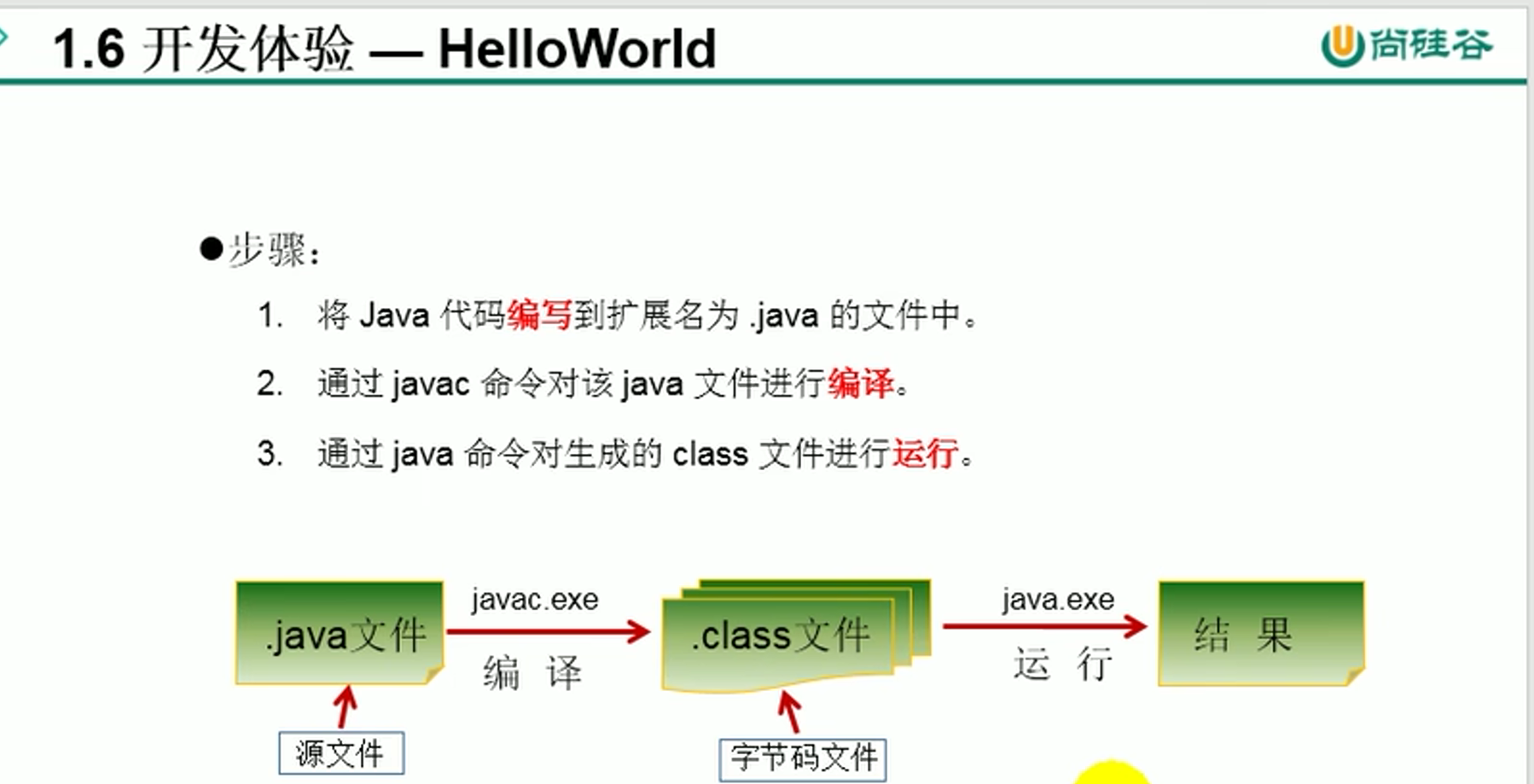### 实践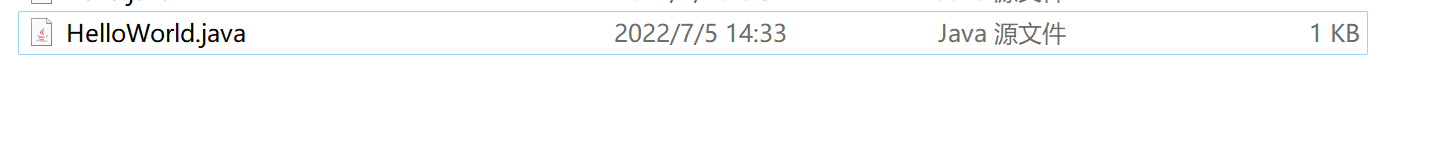``````class quan9i{
}``````

``````class quan9i{
public static void main(String[] args){

}

}``````

``````class quan9i{
public static void main(String[] args){
System.out.println("HelloWorld");
}

}``````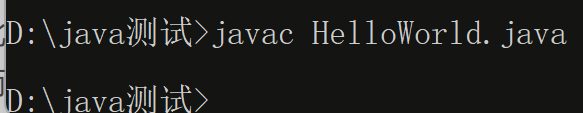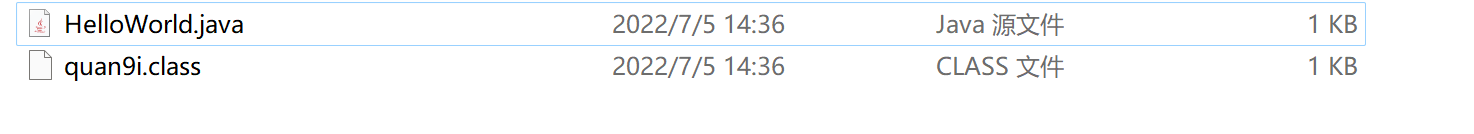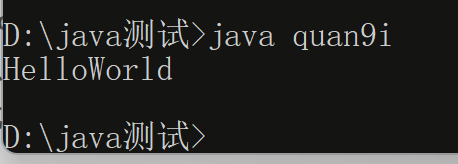### 一点小trick

java语言是严格区分大小写的，但是我们的windows是不区分大小写的，因此我们在将源文件进行编译的时候，其实大写还是小写都是可以成功编译的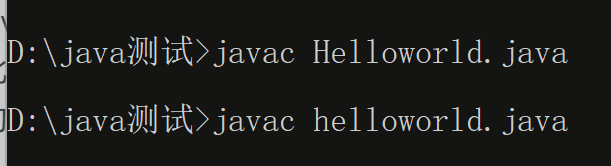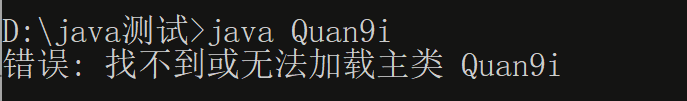### 注意事项

`` System.out.println("HelloWorld");``

``System.out.print("HelloWorld");``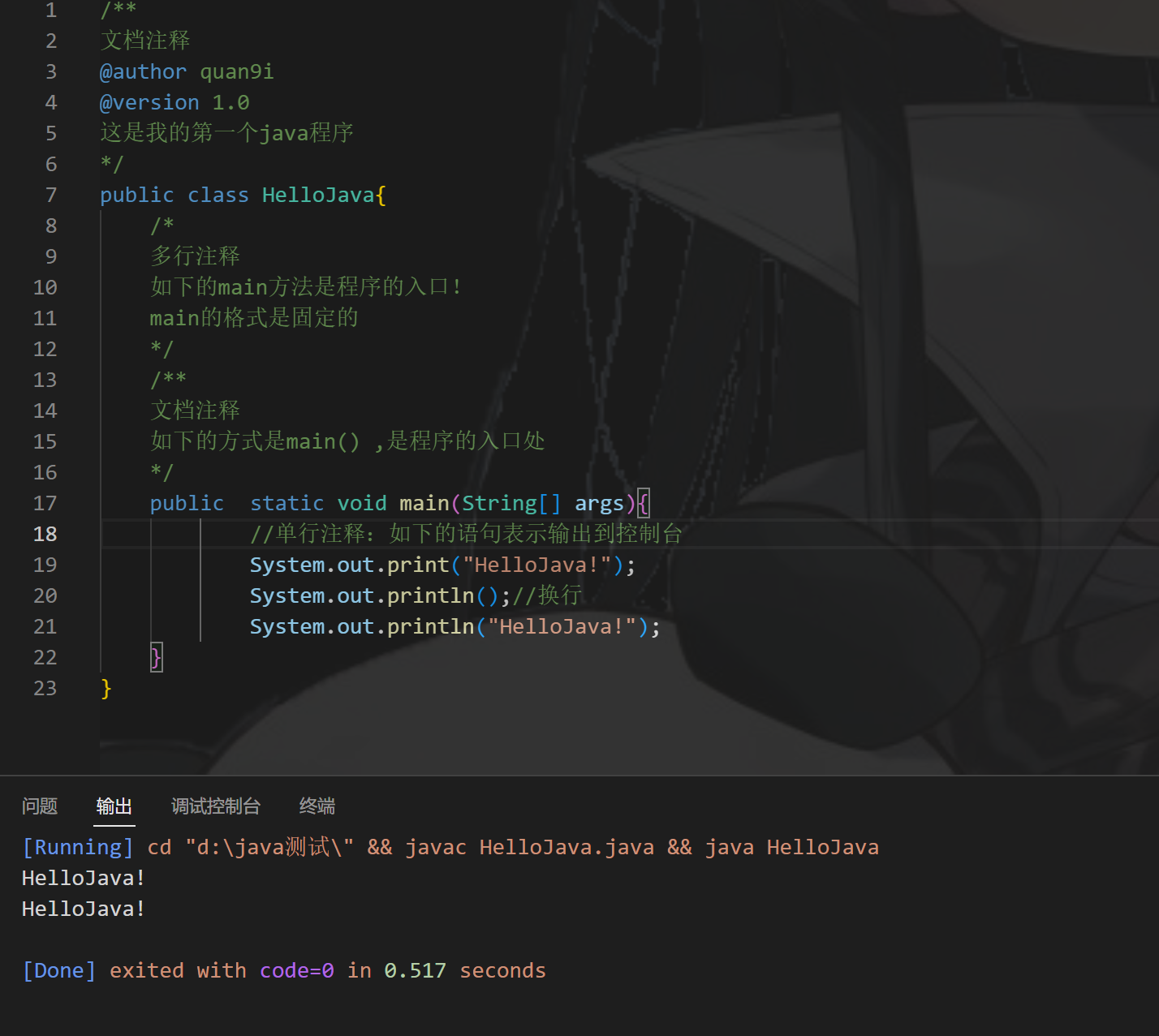## 注释

java 语言中的注释有三种格式

``````1、单行注释
2、多行注释
3、文档注释（java独有）``````

``````1、 //
2、 /*
*/
3、
/**
*/``````

``````对所写的程序进行解释说明，增强代码可读性

### 实践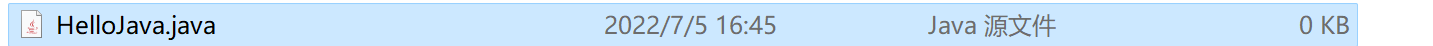``````class HelloJava{
public  static void main(String[] args){
System.out.println("HelloJava");
}
}``````

``````/**

@author quan9i
@version 1.0

*/
class HelloJava{
/*
多行注释
如下的main方法是程序的入口！
main的格式是固定的
*/
/**
文档注释
如下的方式是main() ,是程序的入口处
*/
public  static void main(String[] args){
//单行注释：如下的语句表示输出到控制台
System.out.println("HelloJava");
}
}``````

``javadoc -d 名字 -author -version 文件名``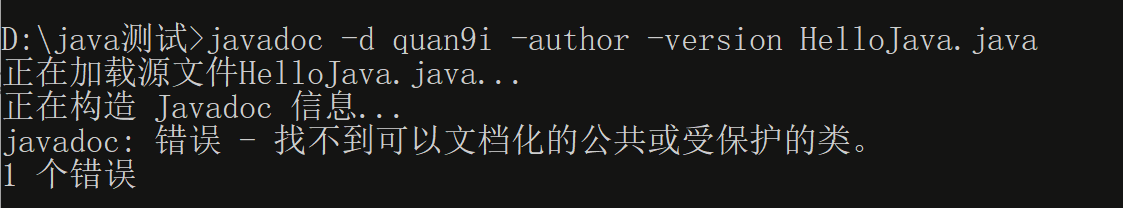``````/**

@author quan9i
@version 1.0

*/
public class HelloJava{
/*
多行注释
如下的main方法是程序的入口！
main的格式是固定的
*/
/**
文档注释
如下的方式是main() ,是程序的入口处
*/
public  static void main(String[] args){
//单行注释：如下的语句表示输出到控制台
System.out.println("HelloJava");
}
}``````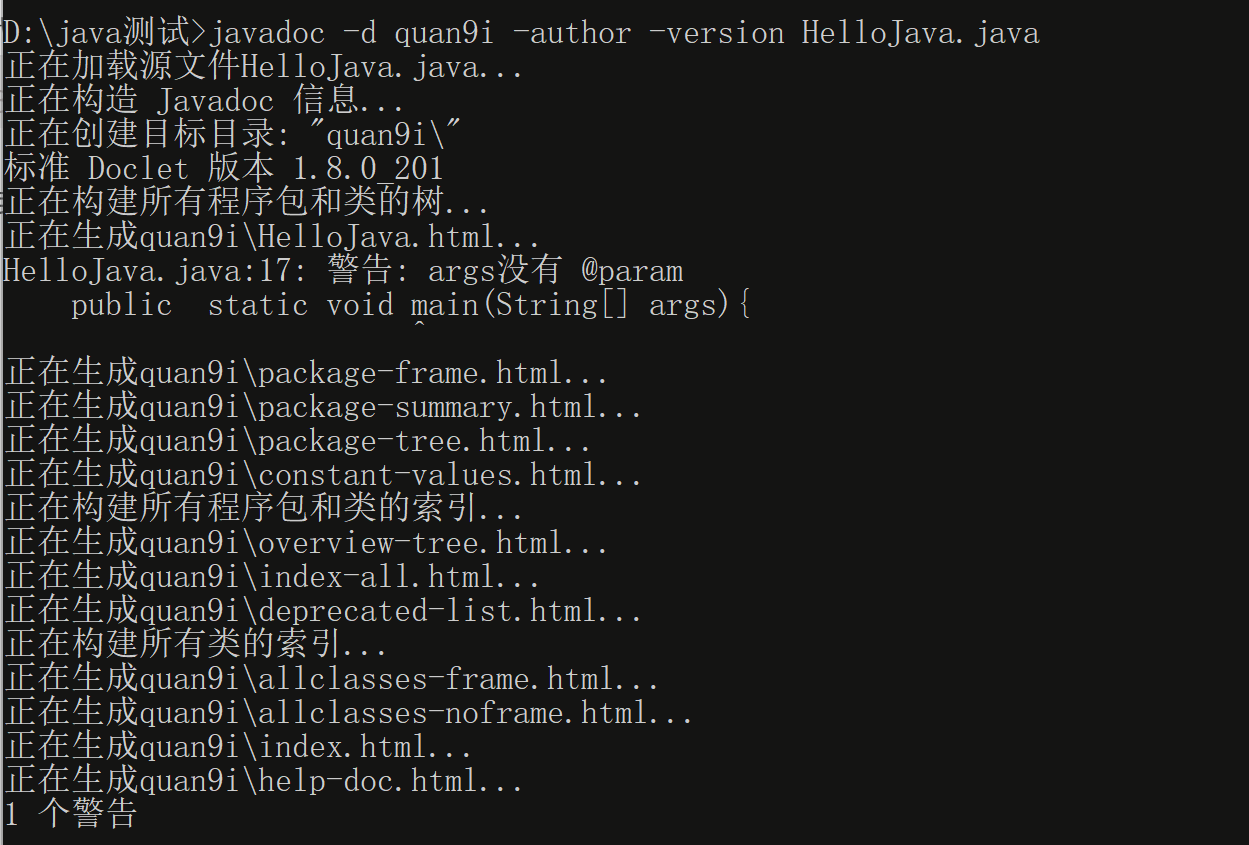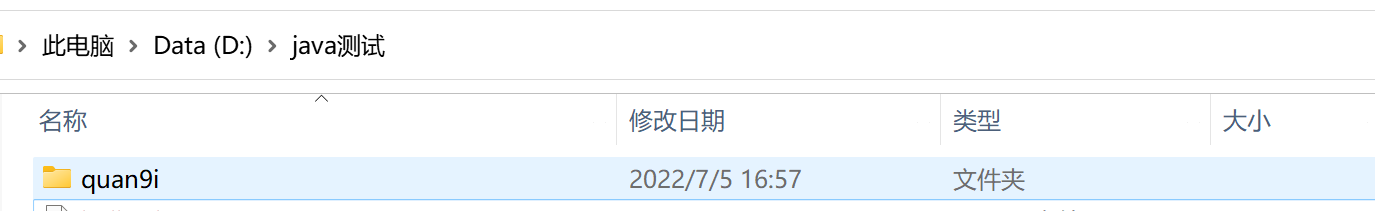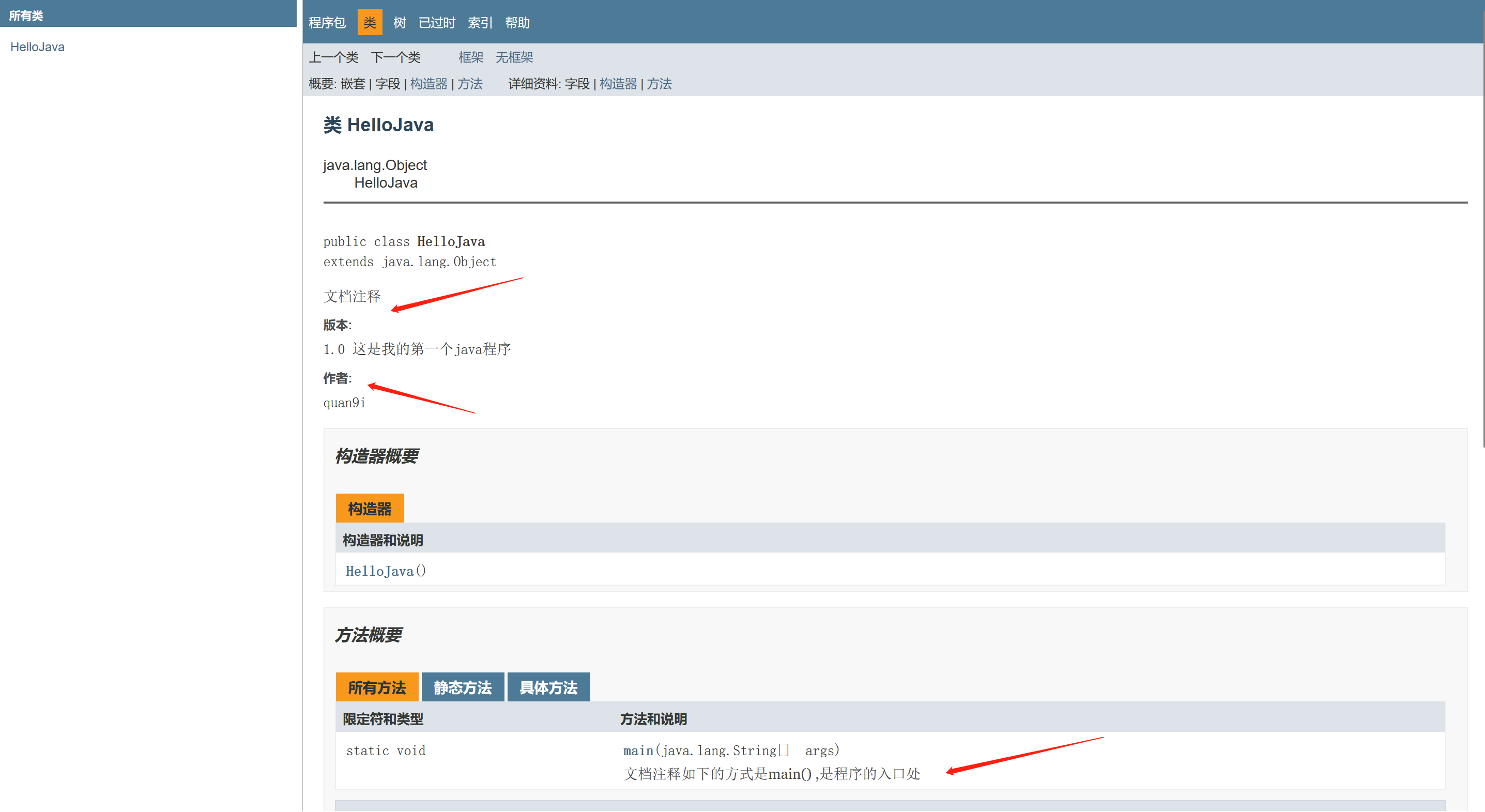### 注意事项

#### 多行注释不可嵌套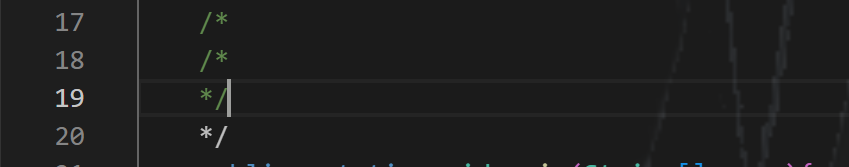## JAVA API

API，全称为`Application Programming Interface`，中文名为`应用程序编程接口`
JAVA语言提供了大量的基础类，因此`ORacle`也为这些基础类提供了相应的API文档，用于告诉开发者如何使用这些类，以及这些类里包含的方法
API入口
https://itmyhome.com/java-api/index.html?overview-summary.html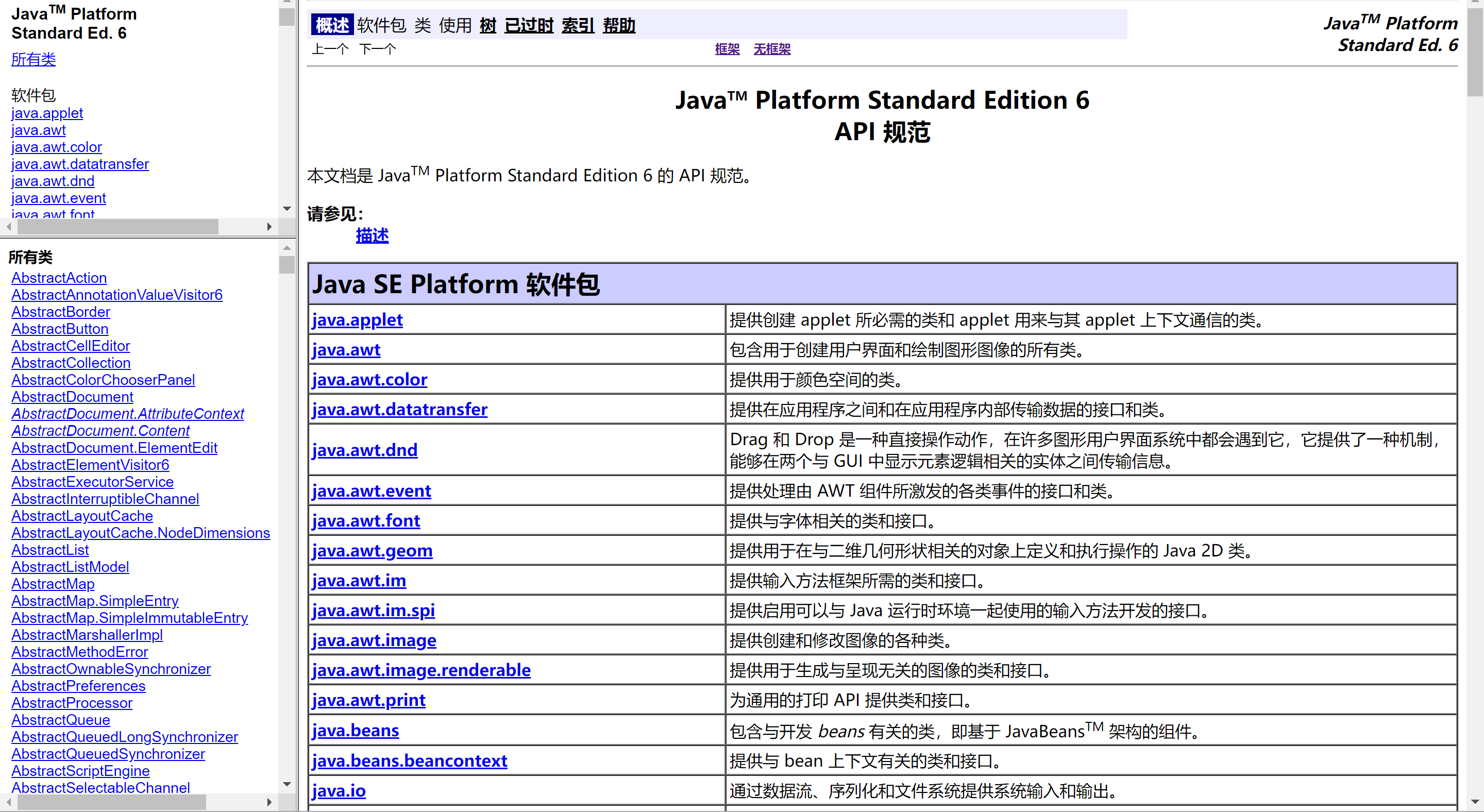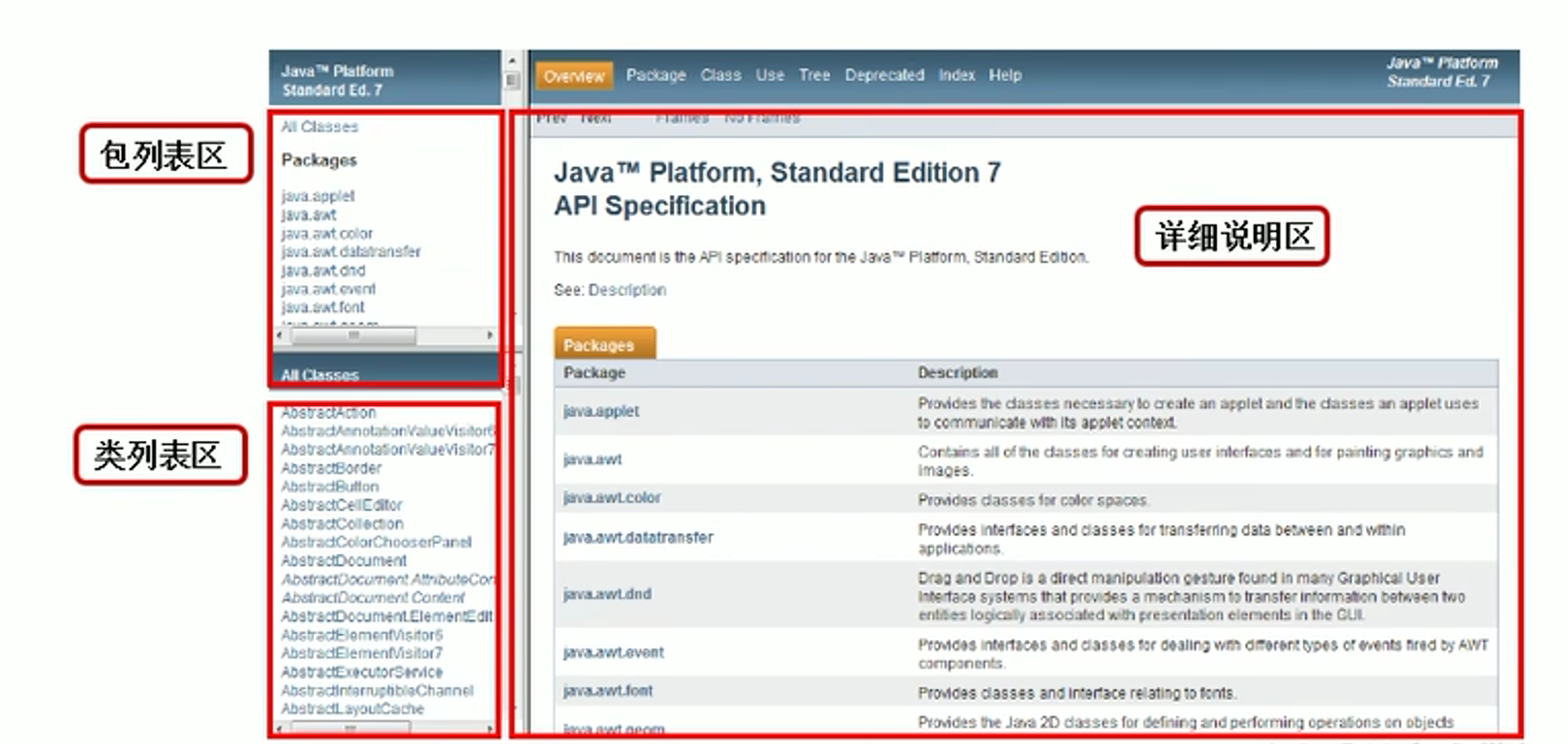### 实践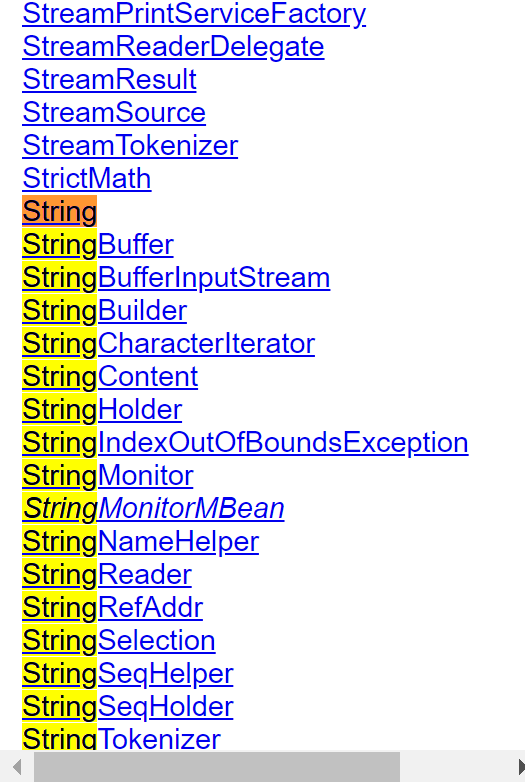# day 02

## 问题

### 问题1

``JDK、JRE、JVM三者之间的关系，以及JDK、JRE包含的主要结构有哪些``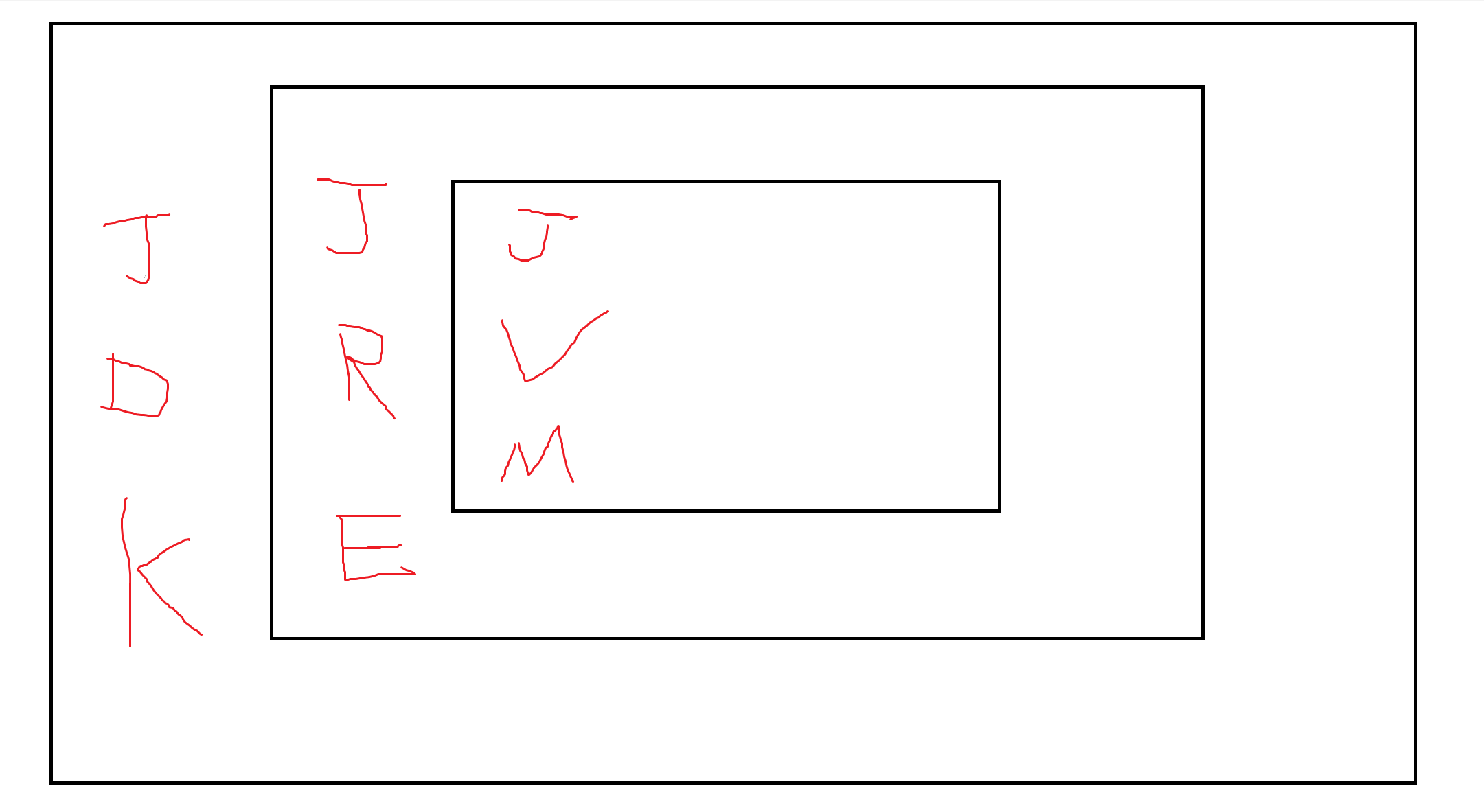JRE=JVM+Java核心类库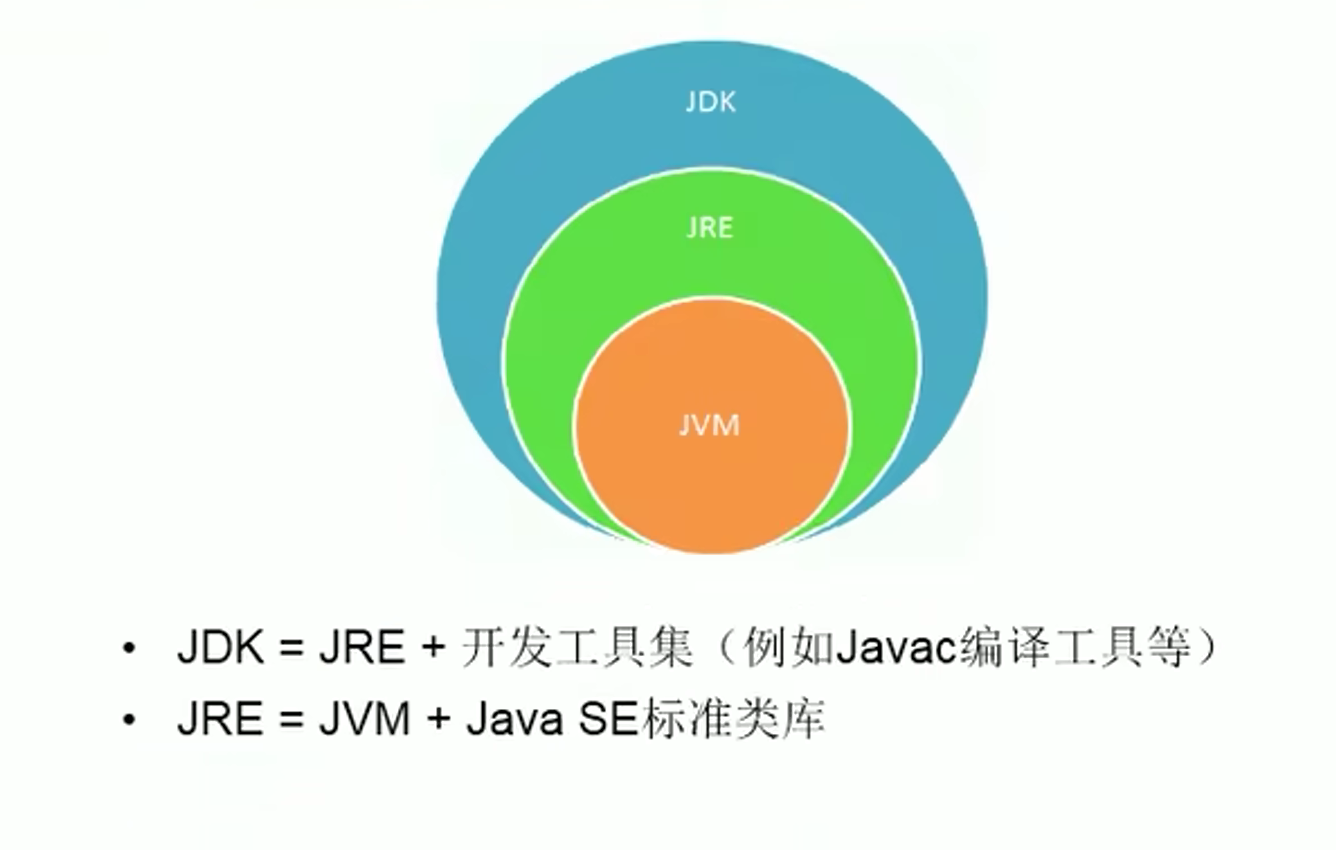### 问题2

``为什么要配置path环境变量``

### 问题3

``````cd //切换目录
rd //删除目录
md //创建目录
dir //列出目录
del //删除文件``````

### 问题4

``````姓名:王大锤

``````class man{
public static void main(String args[]){
System.out.println("姓名:王大锤");
System.out.println();
System.out.println("性别：男");
System.out.print("住址:花果山");

}
}``````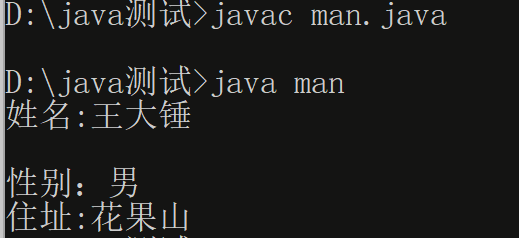## JAVA语言特点

1、面向对象性

``````两个要素：类、对象

2、健壮性

``````1、去除了C语言中的指针
2、自动的垃圾回收机制``````

3、跨平台性

``一次编译，到处运行``

## 关键字与保留字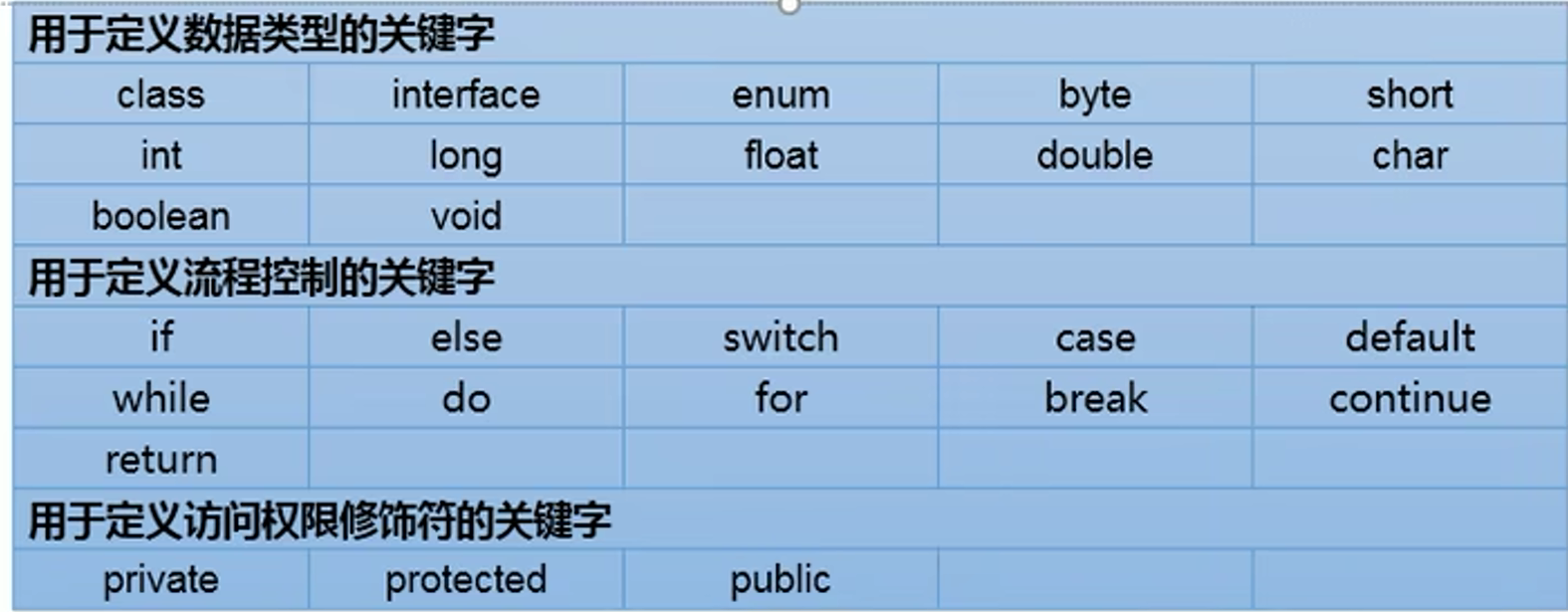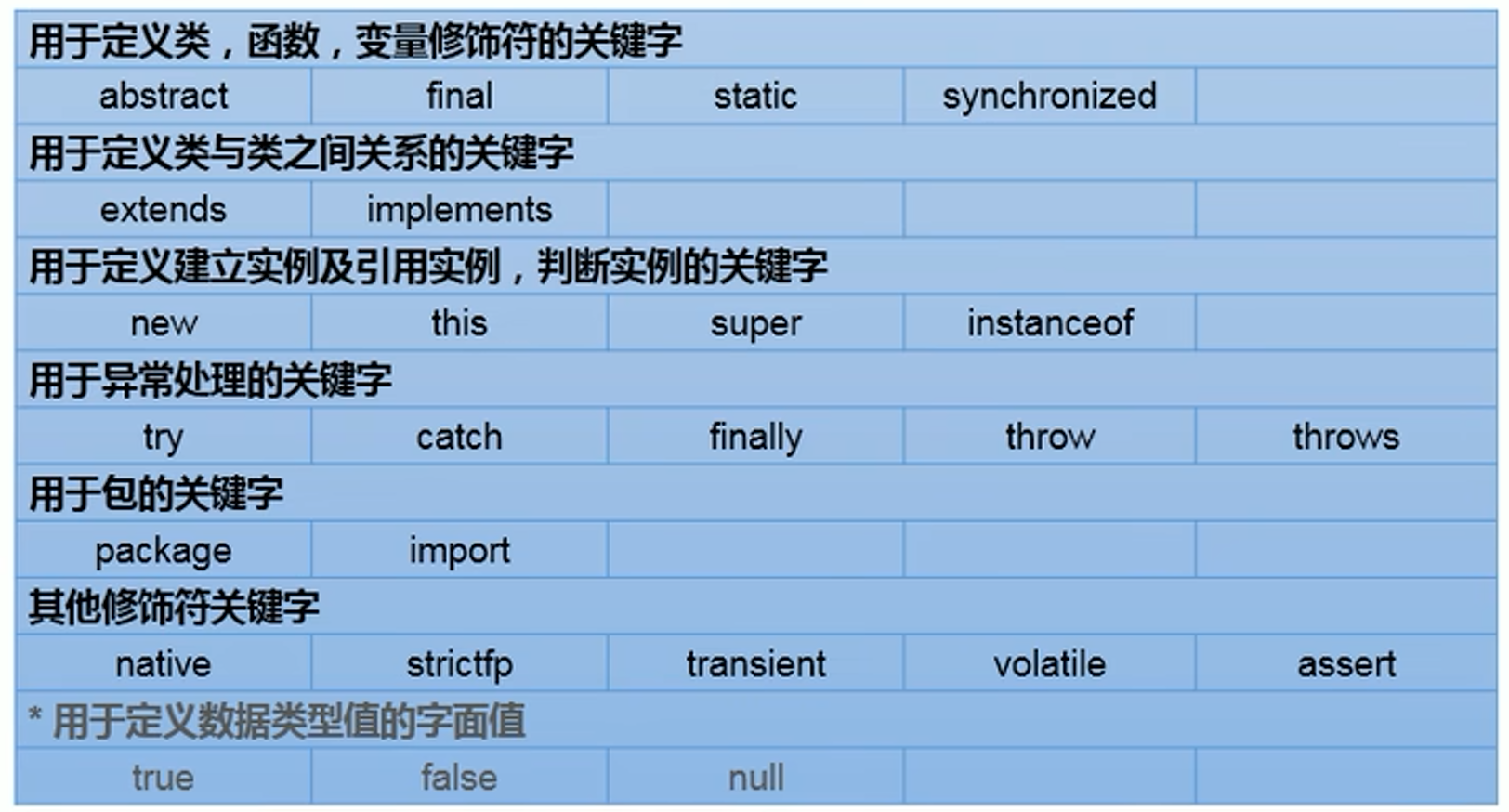Java 保留字列表 (依字母排序 共14组) :
Java保留字是指现有Java版本尚未使用 但以后版本可能会作为关键字使用。
byValue, cast, false, future, generic, inner, operator, outer, rest, true, var， goto ，const,null

## 标识符

### 定义合法标识符规则

``````1、由26个英文字母大小写、0-9、_或\$组成
2、数字不可以开头
3、不可以使用关键字和保留字，但能包含关键字和保留字
4、Java严格区分大小写，长度无限制
5、标识符中不能包含空格``````

### 实践

``````class quan9i{
public static void main(String args[]){
}
}``````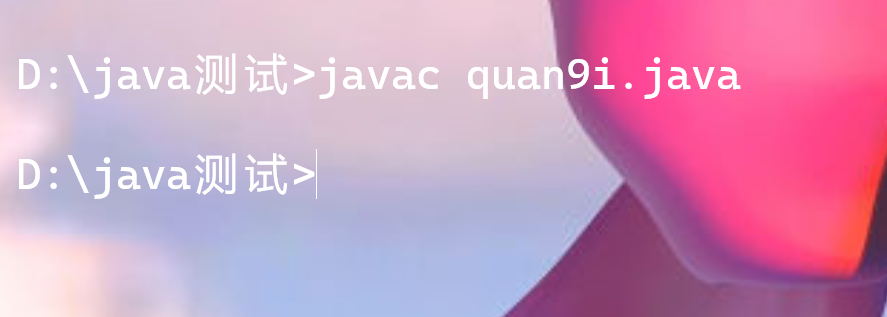``````class 1quan9i{
public static void main(String args[]){

}
}``````

``````class void{
public static void main(String args[]){

}
}``````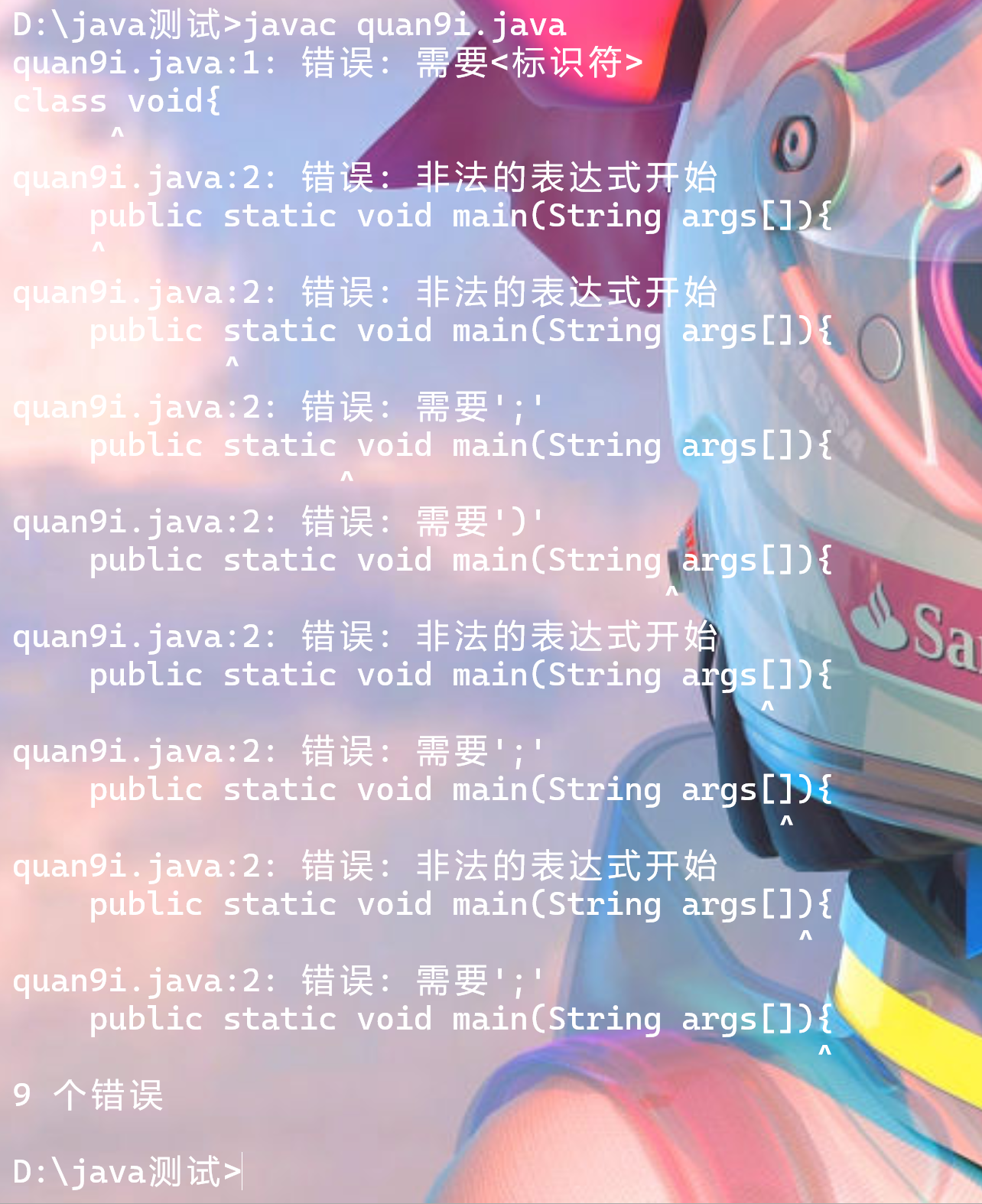``````class void1{
public static void main(String args[]){

}
}``````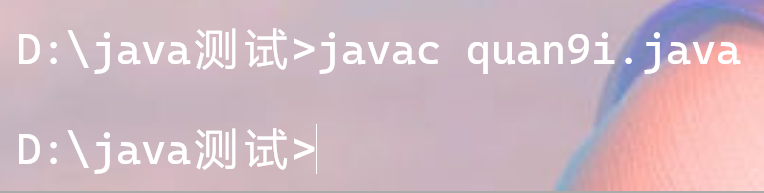`void1`中包含了void关键字，但它不是关键字，因此这里用是没有问题的

``````class Void{
public static void main(String args[]){
}
}``````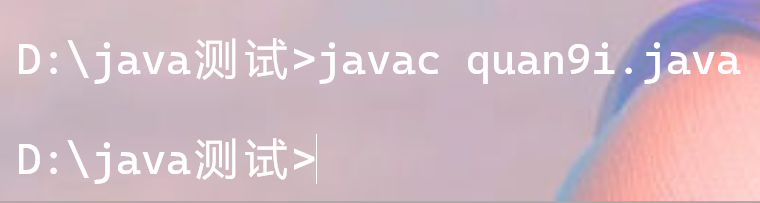``````class Void quan9i{
public static void main(String args[]){

}
}``````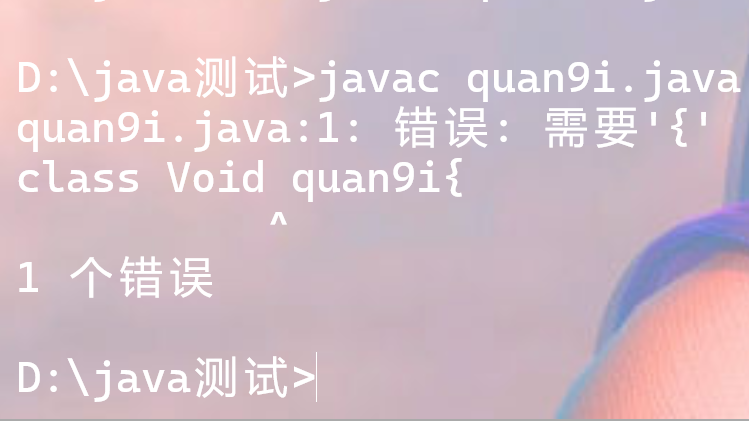### 标识符命名规范

``````class quan9i{
public static void main(String args[]){

}
}``````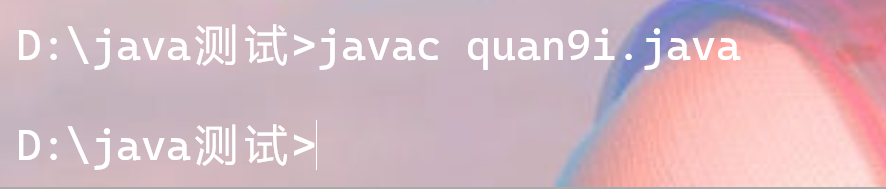## 变量

``用于在内存中存储数据``

``````1、Java中每个变量必须先声明，后使用
2、使用变量名来访问这块区域的数据
3、变量的作用域，其定义在所在的一对{}内
4、变量只有在其作用域中才有效
5、同一个作用域内，不能定义重名的变量``````

### 实践

``````/*

1、java定义变量的格式，数据类型 变量名 = 变量值；
*/
class VariableTest{
public static void main(String[] args){
int myAge = 18;
System.out.println(myAge);
}
}``````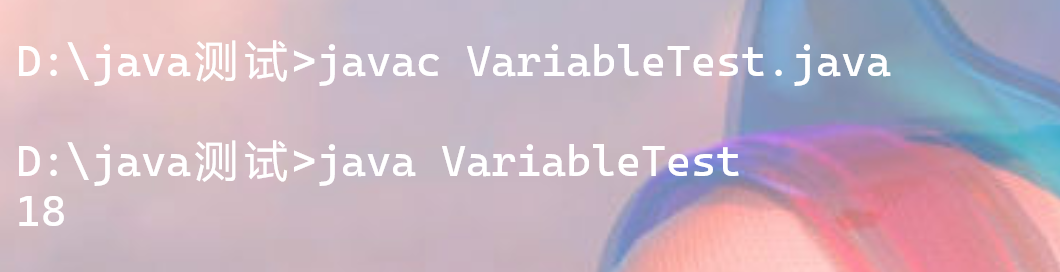``````/*

1、java定义变量的格式，数据类型 变量名 = 变量值；
*/
class VariableTest{
public static void main(String[] args){
int    myAge;
myAge = 18;
System.out.println(myAge);
}
}``````

``````class VariableTest{
public static void main(String[] args){
System.out.println(myAge);
}
public void method(){
int myAge = 1;
}
}``````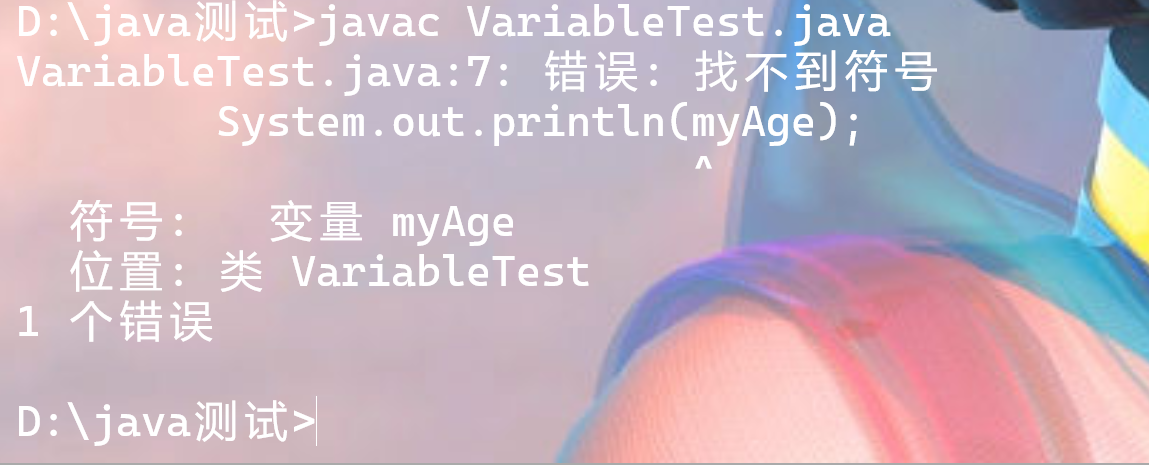``````class VariableTest{
public static void main(String[] args){
int myAge = 18;
System.out.println(myAge);
int myAge = 2;
}
}``````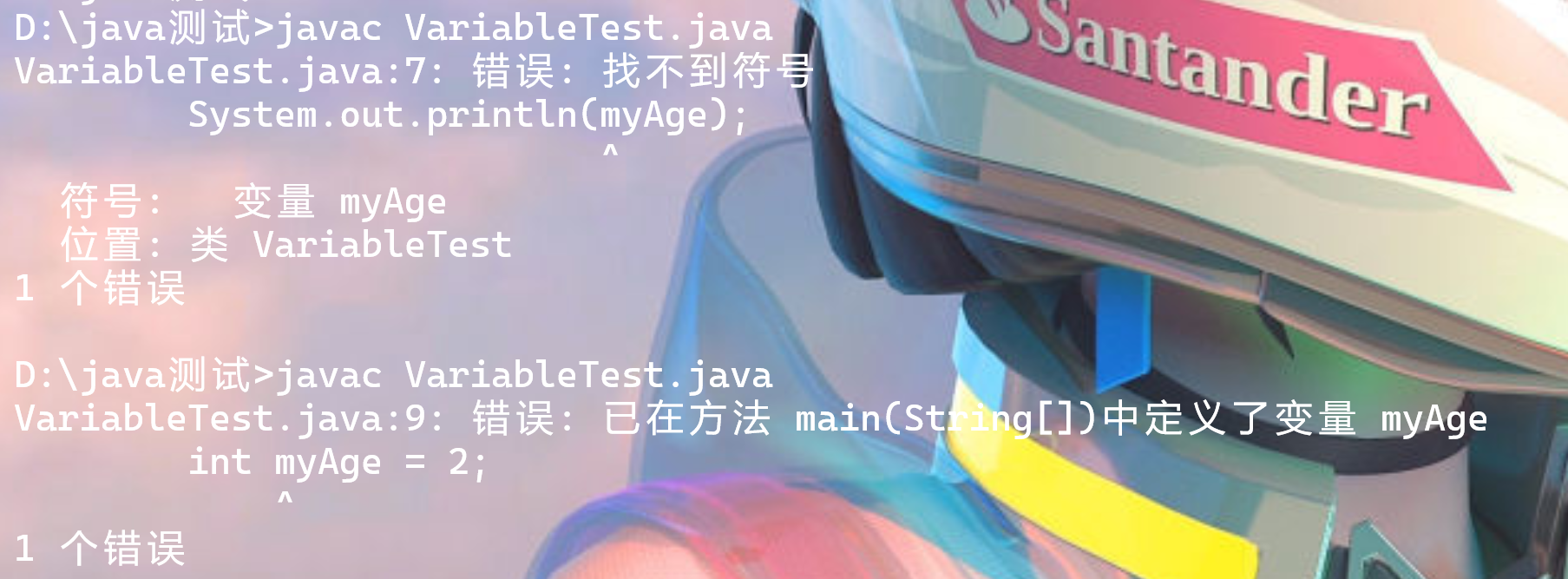### 变量分类

#### 整型变量的使用

byte
short
int
long

##### 测试
``````class VariableTest{
public static void main(String[] args){
byte a1=-127;
byte a2=18;
System.out.println(a1);
System.out.println(a2);
}
}``````
``````class VariableTest{
public static void main(String[] args){
byte a1=-127;
byte a2=18;
short s1=128;
int i1 = 1234;
long l1 = 321231231L;
System.out.println(i1);
System.out.println(l1);
}
}``````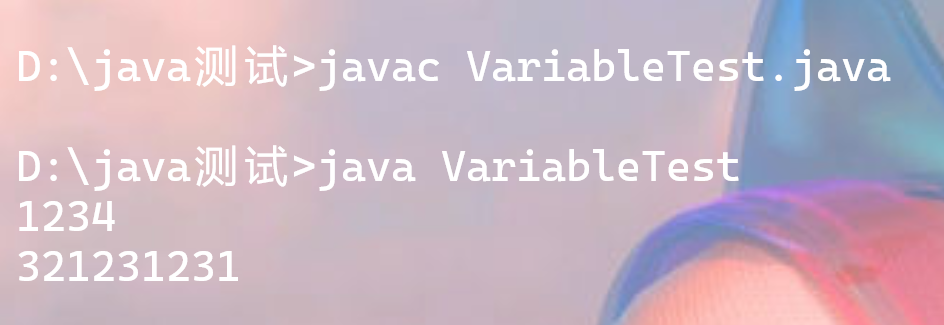# day 03

## 浮点型变量

### 实践

``````class VariableTest{
public static void main(String[] args){
double d1 = 111.11;
float f1 = 22.22;
System.out.println(d1);
System.out.println(f1);
}
}``````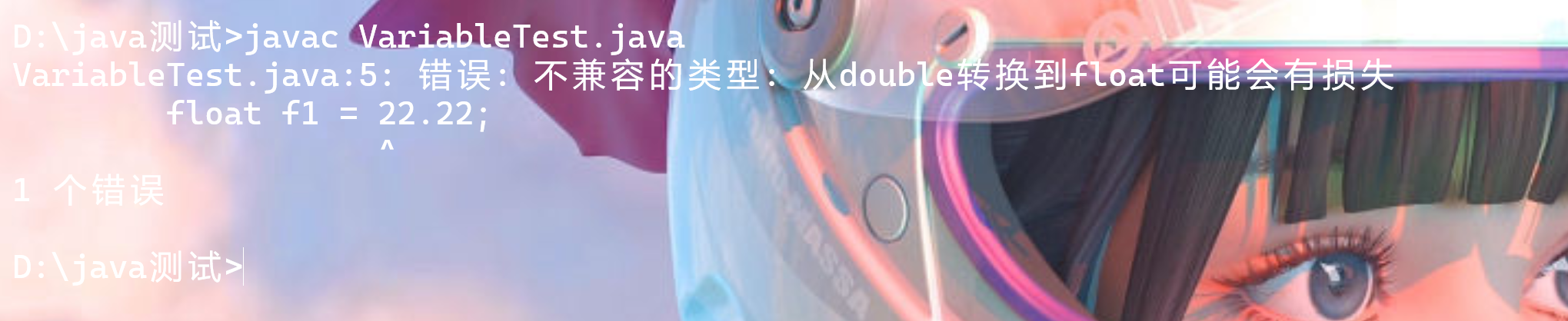``````class VariableTest{
public static void main(String[] args){
double d1 = 111.11;
float f1 = 22.22f;
System.out.println(d1);
System.out.println(f1);
}
}``````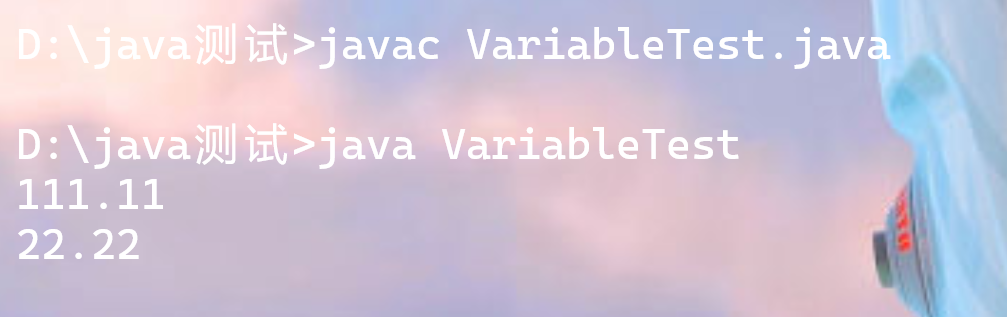## 字符型

char（一字符等于两字节）

``定义char型时，一般用单引号包裹``

1、声明一个字符 例 char a1=’c’;
2、转义字符 例 char a1=’\n’;
3、Unicode字符集。例 char a1=’\u0043′;

### 实践

``````class VariableTest{
public static void main(String[] args){
char c1 = 'a';
char c2 = '1';
char c3 = '\u0043';//字符c
char c4 = '\n';
System.out.println(c1);
System.out.println(c2);
System.out.println(c3);
System.out.print("Hello" + c4);
System.out.println("World");

}
}``````

## 布尔型变量

### 实践

``````class VariableTest{
public static void main(String[] args){
boolean a1=true;
if(a1){
System.out.print("你不可以参加\"单身\"派对了!!!\n很遗憾。");
}
else{
System.out.print("你可以多参加几个\"单身\"派对来交个女朋友！！！");
}

}
}``````

## 数据类型转换

``````（1）占用字节较少的数据类型转换成字节数较多的数据类型；

（2）字符类型会转换成int类型；

（3）int类型会转换成float类型；

（4）若表达式中其中一个类型为double，另一个操作数也会转double；

（5）布尔类型不能转换为其它类型；``````

### 实践

``````class VariableTest{
public static void main(String[] args) {
int i = 10;
long a = 3;
float c =  i/a;
double d = c + a;
System.out.println(i);
System.out.println(a);
System.out.println(c);
System.out.println(d);

}
}``````

# day 04

## 强制类型转换

### 实践

``````class VariableTest{
public static void main(String[] args) {
long l = 123123;
System.out.println(l);
long l1 = 21332423235234123;
System.out.println(l1);
}
}``````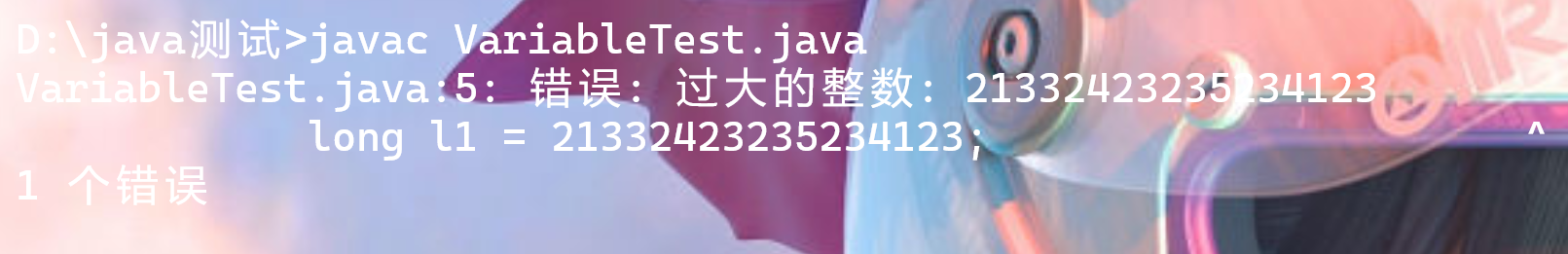``````class VariableTest{
public static void main(String[] args) {
long l = 123123;
System.out.println(l);
long l1 = 21332423235234123L;
System.out.println(l1);
}
}``````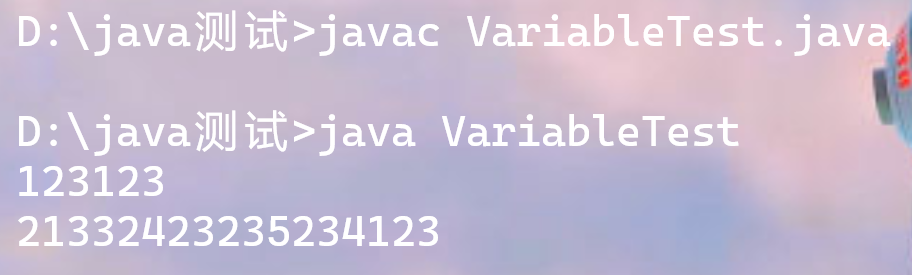``````class VariableTest{
public static void main(String[] args) {
byte b = 12;
byte b1 = b + 1;

}
}``````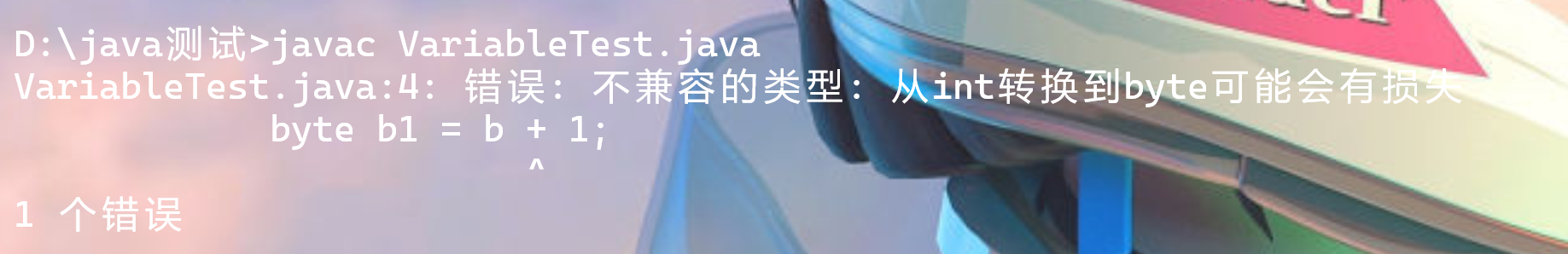``````class VariableTest{
public static void main(String[] args) {
float b = 12;
float b1 = b + 12.2;

}
}``````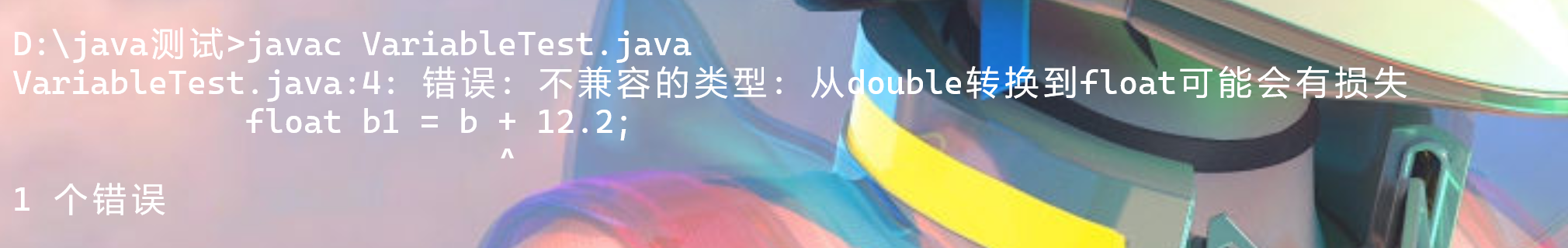``````class VariableTest{
public static void main(String[] args) {
int a = 1;
short b = (short)a;
System.out.println(b);
}
}``````

## String类变量

### 实践

``````class VariableTest{
public static void main(String[] args) {
String s1="Hello World";
System.out.println(s1);
String s2 = "a";
String s3 = "" ;
int number = 666;
String cj="成绩:";
}
}``````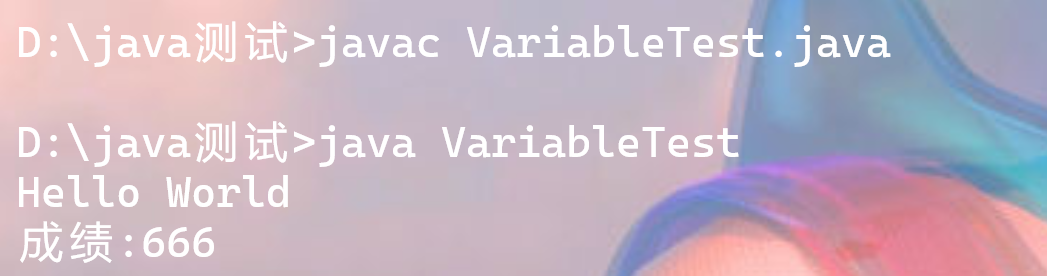``````class VariableTest{
public static void main(String[] args) {
char a = 'a';
int b = 10;
String c = "Hello World";
System.out.println(a+b+c);
System.out.println(a+(b+c));
System.out.println((a+b)+c);
}
}``````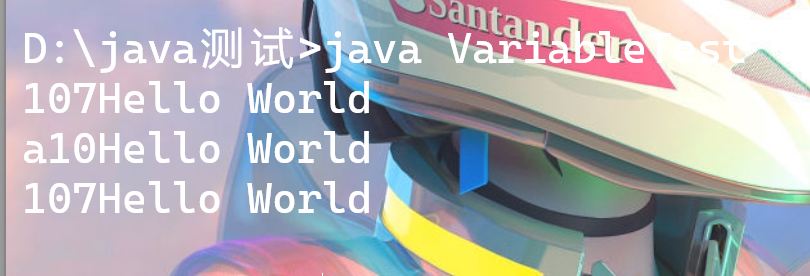``````class VariableTest{
public static void main(String[] args) {
System.out.println('*'+'\t'+'*');
System.out.println('*'+"\t"+'*');
System.out.println('*'+("\t"+'*'));
}
}``````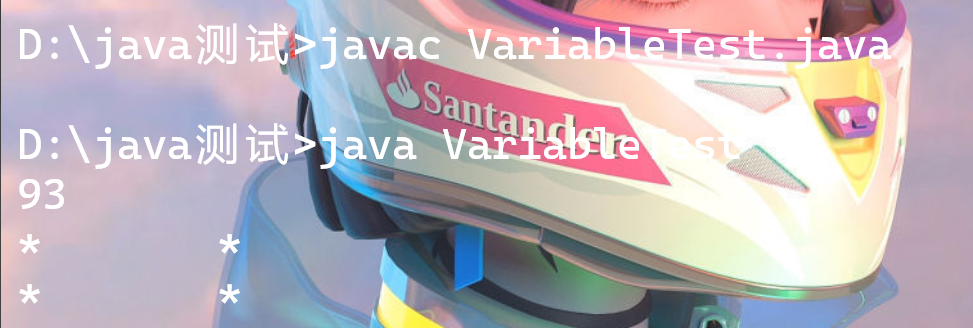## 进制表示方式

### 实践

``````class VariableTest{
public static void main(String[] args) {
int num1 = 0b110;
int num2 = 110;
int num3 = 0110;
int num4 = 0x110;
System.out.println("num1 = "+num1);
System.out.println("num2 = "+num2);
System.out.println("num3 = "+num3);
System.out.println("num4 = "+num4);
}
}``````

# day 05

## 算术运算符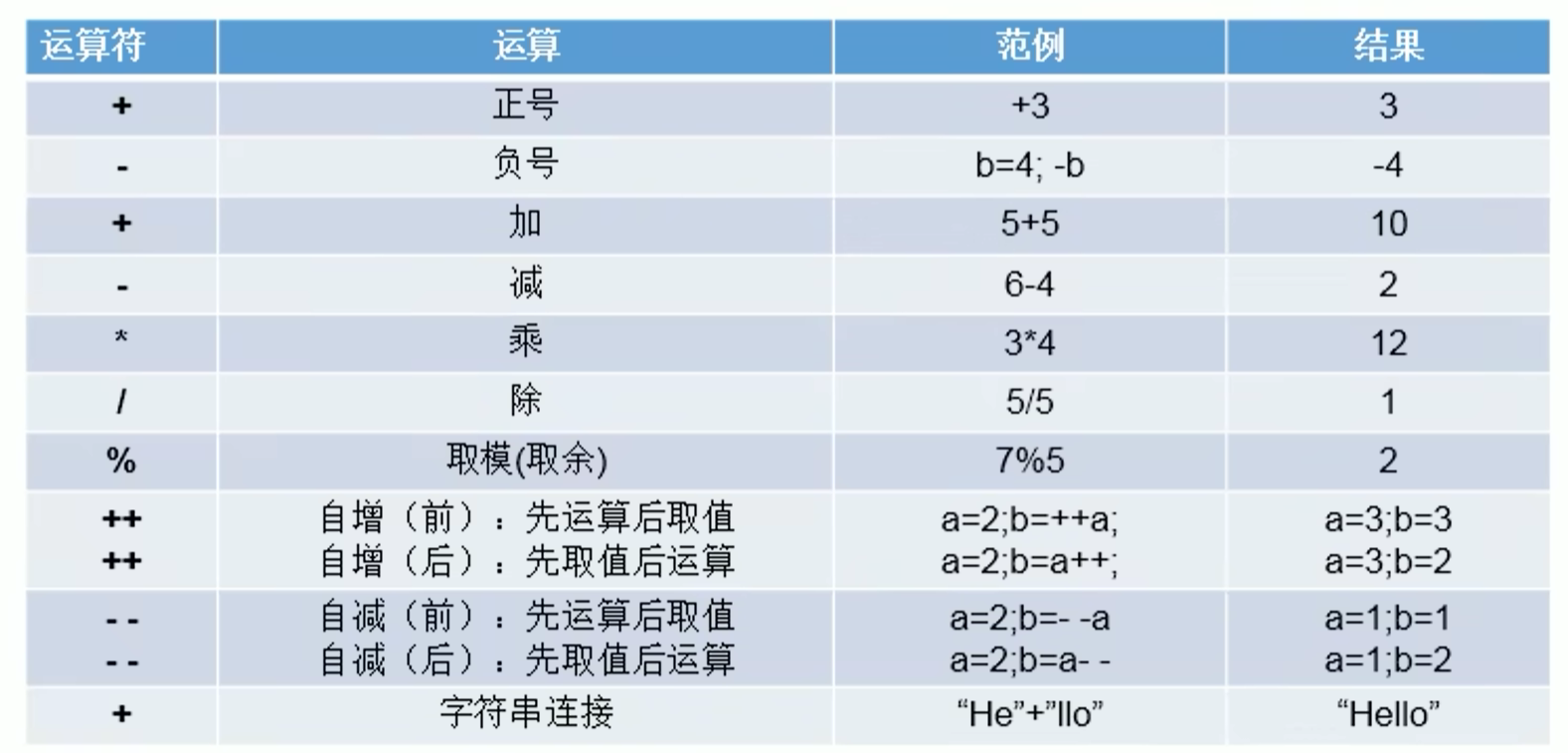### 实践

``````class VariableTest{
public static void main(String[] args) {
int a1 = 13;
int a2= 2;
double b1 = 13/2;
System.out.println(a1%a2);
System.out.println(b1);
System.out.println(-13%2);
System.out.println(13%-2);
System.out.println(-13%-2);
}
}``````
``````class VariableTest{
public static void main(String[] args) {
int a1 = 10;
int b1 = a1++;//先取值后运算，等同于b1=a1;a1=a1+1;
System.out.println(a1);
System.out.println(b1);
int a2 = 10;
int b2 = ++a2;//先运算后取值，等同于a2=a2+1;b2=a2;
System.out.println(a2);
System.out.println(b2);
int a3 = 10;
int b3 = a3--;
System.out.println(a3);//先取值后运算，等同于b3=a3;a3=a3-1##;
System.out.println(b3);
int a4 = 10;
int b4 = --a4;//先运算后赋值，等同于a4=a4-1;b4=a4;
System.out.println(a4);
System.out.println(b4);
}
}``````

### 问题

``````class VariableTest{
public static void main(String[] args) {
int a = 187;
int bai = a /100;
int shi = a %100 /10;
int ge = a %10;
System.out.println("百位:"+bai);
System.out.println("十位:"+shi);
System.out.println("个位:"+ge);

}
}``````

## 比较运算符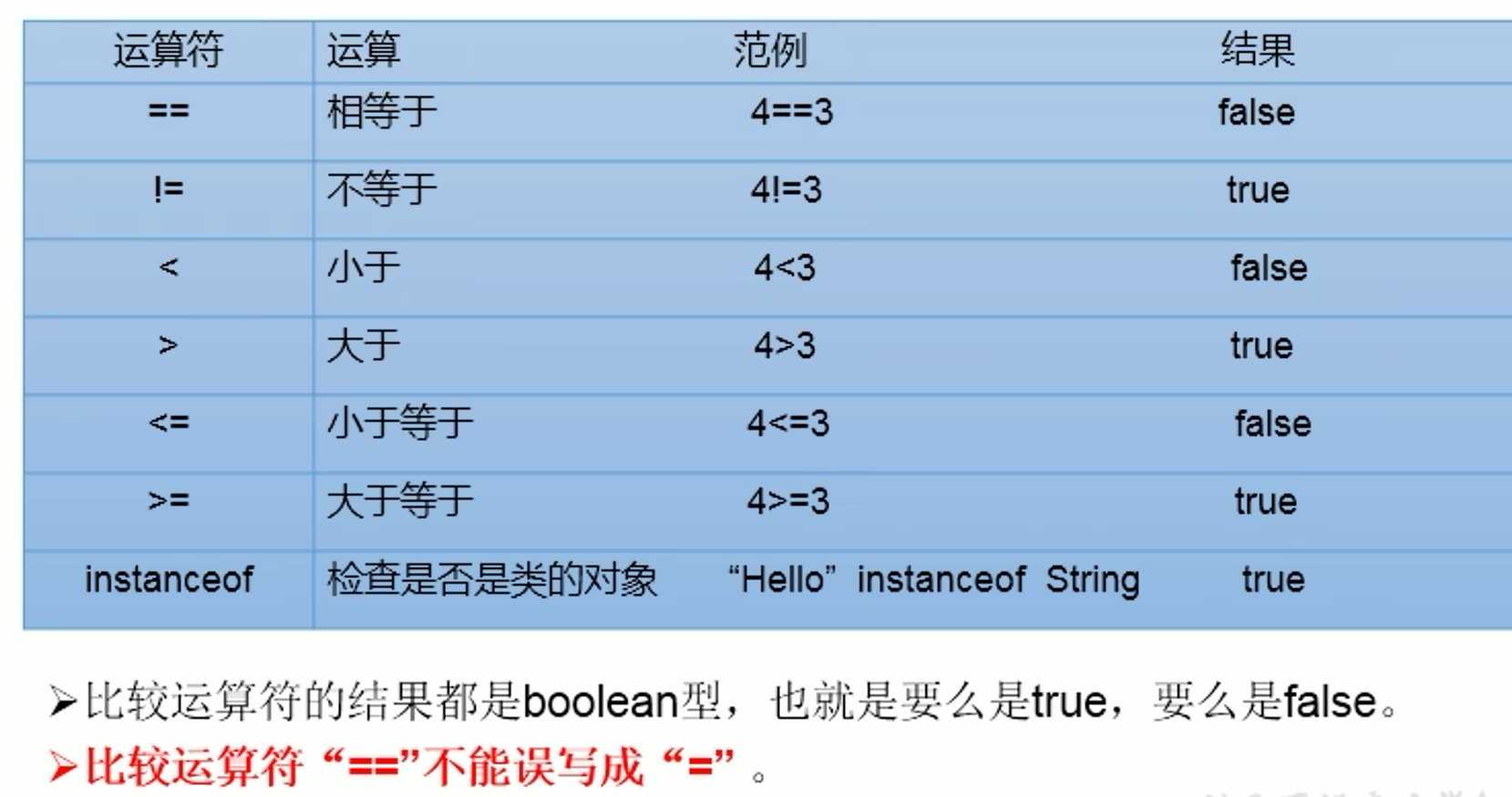## 赋值运算符

``````+=
-=
%=
/=
*=``````

### 实践

``````class VariableTest{
public static void main(String[] args) {
int num1 =10;
num1 +=2;
System.out.println(num1);
int num2 =6;
num2 %= 4;
System.out.println(num2);
int num3 = 3;
num3 *= 3;
System.out.println(num3);

}
}``````

### 小测验

``````class VariableTest{
public static void main(String[] args) {
int i = 1;
i *=0.1;
System.out.println(i);
i++;
System.out.println(i);

}
}``````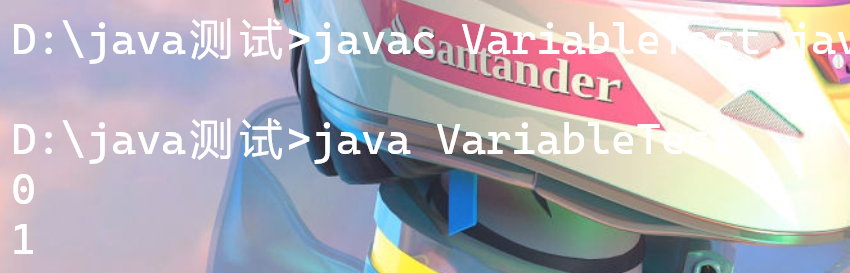``````class VariableTest{
public static void main(String[] args) {
short s = 3;
① s = s + 2;
② s+= s+2
System.out.println(s);
}
}``````

②可以正常编译

# day 06

## 逻辑运算符

``````&
|
!
&&
||
^ ``````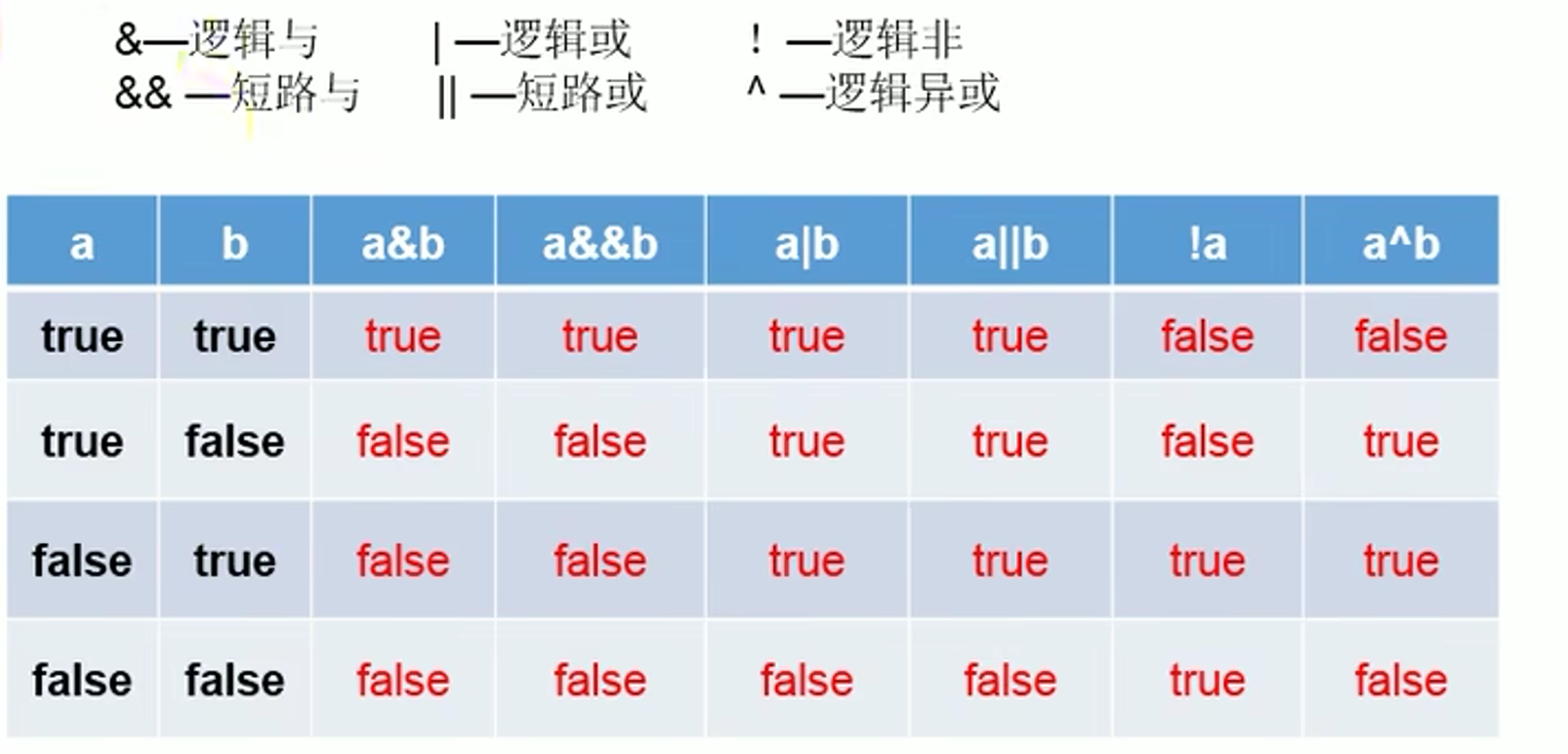### 实践

``````class VariableTest{
public static void main(String[] args) {
boolean b1=false;
int a1=10;
if(b1 & (a1++>0)){
System.out.println("我现在在北京");
}
else{
System.out.println("我现在在南京");
}
System.out.println("a1 = "+a1);

boolean b2=false;
int a2=10;
if(b2 && (a2++>0)){
System.out.println("我现在在北京");
}
else{
System.out.println("我现在在南京");
}
System.out.println("a2 = "+a2);
}
}``````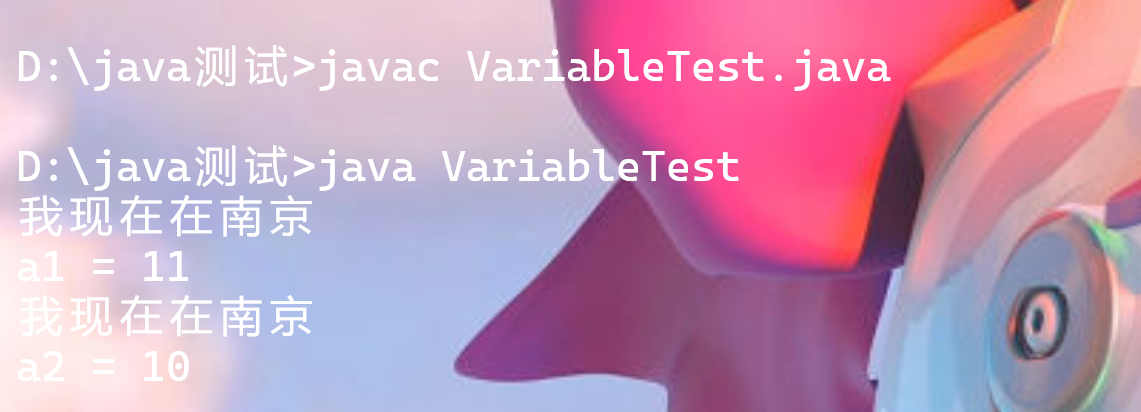``````class VariableTest{
public static void main(String[] args) {
boolean b1=true;
int a1=10;
if(b1 | (a1++>0)){
System.out.println("我现在在北京");
}
else{
System.out.println("我现在在南京");
}
System.out.println("a1 = "+a1);

boolean b2=true;
int a2=10;
if(b2 || (a2++>0)){
System.out.println("我现在在北京");
}
else{
System.out.println("我现在在南京");
}
System.out.println("a2 = "+a2);
}
}``````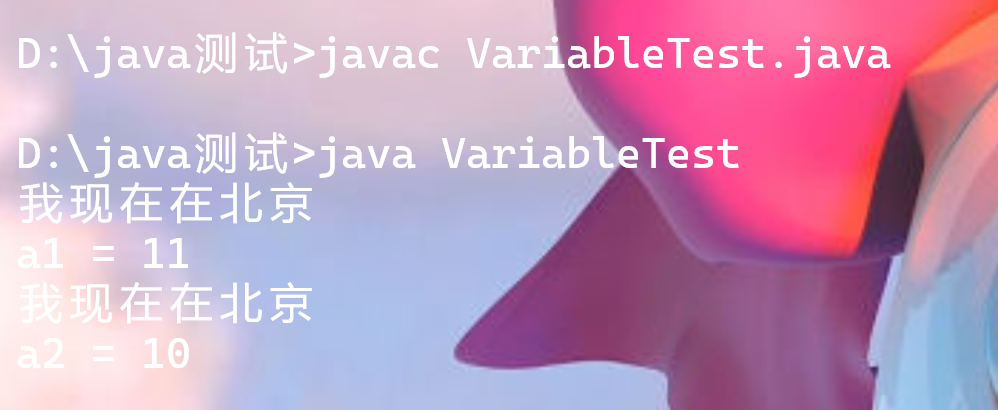## 位运算符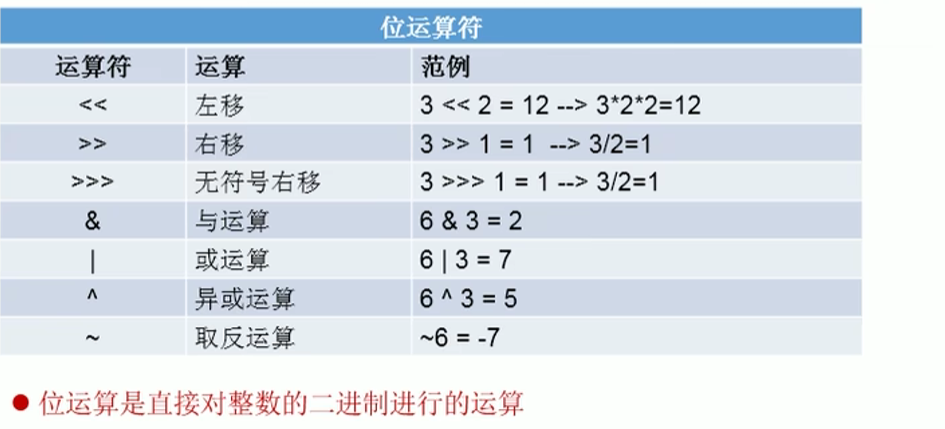`````` 12 8 4 2 1  结果
0 0 0 1 1 | 3
左移二位
0 1 1 0 0 | 12
右移一位
0 0 0 0 1 | 1``````

`````` 12 8 4 2 1  结果
0 0 1 1 0 | 6
0 0 0 1 1 | 3
经过&运算
0 0 0 1 0 | 2
经过|运算
0 0 1 1 1 | 7
经过^运算（相同取0，不同取1）
0 0 1 0 1 | 5``````

``````位运算符操作的都是整型的数据
<<:在一定范围内，每左移1位，相当于 *2
>>:在一定范围内，每右移1位，相当于 /2``````

### 实践

``````class VariableTest{
public static void main(String[] args) {
int  i = 20;
System.out.println("i<<2="+(i<<2));
System.out.println("i>>2="+(i>>2));
}
}``````

## 三元运算符

``a?b:c``

### 实践

``````class VariableTest{
public static void main(String[] args) {
int a = 12;
int b = 30;
int c = -40;
int max1 = (a>b)?a:b;
int max2 = (max1>c)?max1:c;
System.out.println("三个数中的最大值为:"+max2);
}
}``````

# day 07

## if – else流程控制

``````if(条件1){
xxx
}
else{
xxx
}``````

``````if(条件1){
xxx
}
else if(条件2){
xxx
}
else{
xxx
}``````

### 实践

``````class VariableTest{
public static void main(String[] args) {
int a = 12;
int b = 30;
if(a>b){
System.out.println("最大值为:" + a);
}
else{
System.out.println("最大值为:" + b);
}
}
}``````
``````class VariableTest{
public static void main(String[] args) {
int a = 12;
int b = 30;
if(a>b){
System.out.println("最大值为:" + a);
}
else if(a<b){
System.out.println("最大值为:" + b);
}
else{
System.out.println("两者无法确定最大值");
}
}
}``````

## scanner

``````1:导包：import java.util.Scanner;
2:Scanner的实例化:Scanner scan = new Scanner(System.in);
3:调用Scanner类的相关方法，来获取指定类型的变量。``````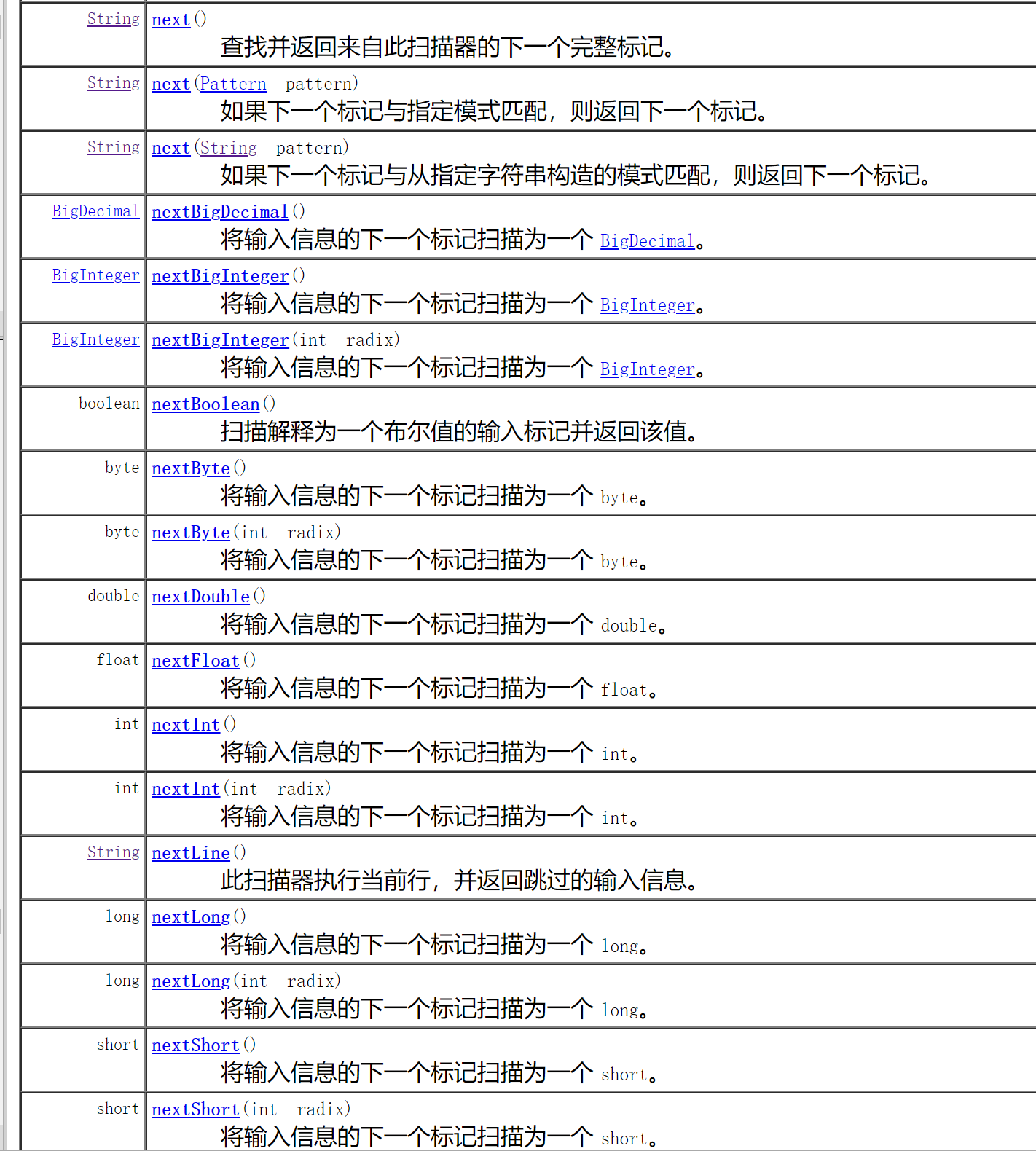``````import java.util.Scanner;

class VariableTest{
public static void main(String[] args) {
Scanner scan = new Scanner(System.in);
int num = scan.nextInt();
System.out.println(num);
}
}``````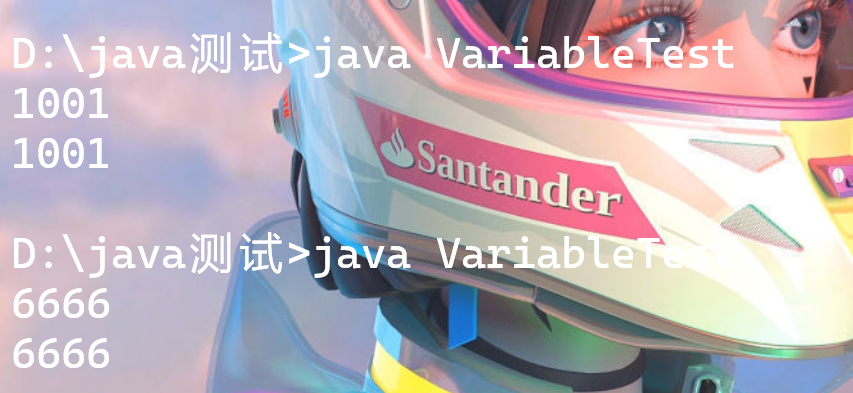### 实践

``````/*

1:导包：import java.util.Scanner;
2:Scanner的实例化:Scanner scan = new Scanner(System.in);
3:调用Scanner类的相关方法，来获取指定类型的变量。
*/
import java.util.Scanner;

class VariableTest{
public static void main(String[] args) {
Scanner scan = new Scanner(System.in);
System.out.println("请输入您的姓名");
String name = scan.next();
System.out.println(name);

System.out.println("请输入您的年龄");
int age = scan.nextInt();
System.out.println(age);

System.out.println("请输入您的体重");
double weight = scan.nextDouble();
System.out.println(weight);

System.out.println("你是否对我有好感?(true/false)");
boolean isgood= scan.nextBoolean();
System.out.println(isgood);

//对于char型的获取，Scanner没有提供相关方法，只能获取一个字符串
System.out.println("请输入您的性别(男/女)");
String xingbie = scan.next();
char xb = xingbie.charAt(0);
}
}``````

# day 08

## switch – case

``````switch(表达式){
case 常量1:
执行语句1；
//break;(可写可不写，写的话就跳出去了，不写继续往后执行)
case 常量2
执行语句2:
//break;
...
default:(case中没有对应的常量时执行)
执行语句n;
//break;``````

### 实践

``````import java.util.Scanner;

class VariableTest{
public static void main(String[] args) {
Scanner scan = new Scanner(System.in);
System.out.println("请输入常量");
int number = scan.nextInt();
switch(number){
case 0:
System.out.println("zero");

case 1:
System.out.println("one");
break;
case 2:
System.out.println("two");
break;
default:
System.out.println("other");
break;
}
}
}``````

## for循环

``````for(变量;条件;自增){
循环体
}``````

### 实践

``````class VariableTest{
public static void main(String[] args) {
for(int i=0;i<=3;i++){
System.out.println("quan9i");
}
}
}``````

## while循环

``````while(循环条件){
循环体
}``````

### 实践

``````class VariableTest{
public static void main(String[] args) {
int i=1;
while(i<100){
if(i%2==0){
System.out.println(i);
}
i++;
}
}
}``````

## do -while 循环

``````do{
循环体
}
while(循环条件);``````

### 实践

``````class VariableTest{
public static void main(String[] args) {
int i=1;
do{
if(i%2==0){
System.out.println(i);
}
i++;
}
while(i<100);
}
}``````

## 发送评论编辑评论

|´・ω・)ノ
ヾ(≧∇≦*)ゝ
(☆ω☆)
（╯‵□′）╯︵┴─┴
￣﹃￣
(/ω＼)
∠( ᐛ 」∠)＿
(๑•̀ㅁ•́ฅ)
→_→
୧(๑•̀⌄•́๑)૭
٩(ˊᗜˋ*)و
(ノ°ο°)ノ
(´இ皿இ｀)
⌇●﹏●⌇
(ฅ´ω`ฅ)
(╯°A°)╯︵○○○
φ(￣∇￣o)
ヾ(´･ ･｀｡)ノ"
( ง ᵒ̌皿ᵒ̌)ง⁼³₌₃
(ó﹏ò｡)
Σ(っ °Д °;)っ
( ,,´･ω･)ﾉ"(´っω･｀｡)
╮(╯▽╰)╭
o(*////▽////*)q
＞﹏＜
( ๑´•ω•) "(ㆆᴗㆆ)

Emoji

...